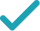Type a math problemSolve for x (complex solution)Steps Using the Quadratic Formula
Steps for Completing the Square
Use binomial theorem to expand .
Consider . Multiplication can be transformed into difference of squares using the rule: . Square .
Subtract from both sides.
Combine and to get .
This equation is in standard form: . Substitute for , for , and for in the quadratic formula, .
Square .
Multiply times .
Multiply times .
Take the square root of .
The opposite of is .
Multiply times .
Now solve the equation when is plus. Add to .
Divide by .
Now solve the equation when is minus. Subtract from .
Divide by .
The equation is now solved.
GraphGiving is as easy as 1, 2, 3
Get 1,000 points to donate to a school of your choice when you join Give With Bing
16x^{2}-8x+1=\left(x-1\right)\left(x+1\right)
Use binomial theorem \left(a-b\right)^{2}=a^{2}-2ab+b^{2} to expand \left(4x-1\right)^{2}.
16x^{2}-8x+1=x^{2}-1
Consider \left(x-1\right)\left(x+1\right). Multiplication can be transformed into difference of squares using the rule: \left(a-b\right)\left(a+b\right)=a^{2}-b^{2}. Square 1.
16x^{2}-8x+1-x^{2}=-1
Subtract x^{2} from both sides.
15x^{2}-8x+1=-1
Combine 16x^{2} and -x^{2} to get 15x^{2}.
15x^{2}-8x+1+1=0
15x^{2}-8x+2=0
Add 1 and 1 to get 2.
x=\frac{-\left(-8\right)±\sqrt{\left(-8\right)^{2}-4\times 15\times 2}}{2\times 15}
This equation is in standard form: ax^{2}+bx+c=0. Substitute 15 for a, -8 for b, and 2 for c in the quadratic formula, \frac{-b±\sqrt{b^{2}-4ac}}{2a}.
x=\frac{-\left(-8\right)±\sqrt{64-4\times 15\times 2}}{2\times 15}
Square -8.
x=\frac{-\left(-8\right)±\sqrt{64-60\times 2}}{2\times 15}
Multiply -4 times 15.
x=\frac{-\left(-8\right)±\sqrt{64-120}}{2\times 15}
Multiply -60 times 2.
x=\frac{-\left(-8\right)±\sqrt{-56}}{2\times 15}
x=\frac{-\left(-8\right)±2\sqrt{14}i}{2\times 15}
Take the square root of -56.
x=\frac{8±2\sqrt{14}i}{2\times 15}
The opposite of -8 is 8.
x=\frac{8±2\sqrt{14}i}{30}
Multiply 2 times 15.
x=\frac{8+2\sqrt{14}i}{30}
Now solve the equation x=\frac{8±2\sqrt{14}i}{30} when ± is plus. Add 8 to 2i\sqrt{14}\approx 7.483314774i.
x=\frac{4+\sqrt{14}i}{15}
Divide 8+2i\sqrt{14}\approx 8+7.483314774i by 30.
x=\frac{-2\sqrt{14}i+8}{30}
Now solve the equation x=\frac{8±2\sqrt{14}i}{30} when ± is minus. Subtract 2i\sqrt{14}\approx 7.483314774i from 8.
x=\frac{-\sqrt{14}i+4}{15}
Divide 8-2i\sqrt{14}\approx 8-7.483314774i by 30.
x=\frac{4+\sqrt{14}i}{15} x=\frac{-\sqrt{14}i+4}{15}
The equation is now solved.
16x^{2}-8x+1=\left(x-1\right)\left(x+1\right)
Use binomial theorem \left(a-b\right)^{2}=a^{2}-2ab+b^{2} to expand \left(4x-1\right)^{2}.
16x^{2}-8x+1=x^{2}-1
Consider \left(x-1\right)\left(x+1\right). Multiplication can be transformed into difference of squares using the rule: \left(a-b\right)\left(a+b\right)=a^{2}-b^{2}. Square 1.
16x^{2}-8x+1-x^{2}=-1
Subtract x^{2} from both sides.
15x^{2}-8x+1=-1
Combine 16x^{2} and -x^{2} to get 15x^{2}.
15x^{2}-8x=-1-1
Subtract 1 from both sides.
15x^{2}-8x=-2
Subtract 1 from -1 to get -2.
\frac{15x^{2}-8x}{15}=\frac{-2}{15}
Divide both sides by 15.
x^{2}+\frac{-8}{15}x=\frac{-2}{15}
Dividing by 15 undoes the multiplication by 15.
x^{2}-\frac{8}{15}x=\frac{-2}{15}
Divide -8 by 15.
x^{2}-\frac{8}{15}x=-\frac{2}{15}
Divide -2 by 15.
x^{2}-\frac{8}{15}x+\left(-\frac{4}{15}\right)^{2}=-\frac{2}{15}+\left(-\frac{4}{15}\right)^{2}
Divide -\frac{8}{15}\approx -0.533333333, the coefficient of the x term, by 2 to get -\frac{4}{15}\approx -0.266666667. Then add the square of -\frac{4}{15}\approx -0.266666667 to both sides of the equation. This step makes the left hand side of the equation a perfect square.
x^{2}-\frac{8}{15}x+\frac{16}{225}=-\frac{2}{15}+\frac{16}{225}
Square -\frac{4}{15}\approx -0.266666667 by squaring both the numerator and the denominator of the fraction.
x^{2}-\frac{8}{15}x+\frac{16}{225}=-\frac{14}{225}
Add -\frac{2}{15}\approx -0.133333333 to \frac{16}{225}\approx 0.071111111 by finding a common denominator and adding the numerators. Then reduce the fraction to lowest terms if possible.
\left(x-\frac{4}{15}\right)^{2}=-\frac{14}{225}
Factor x^{2}-\frac{8}{15}x+\frac{16}{225}. In general, when x^{2}+bx+c is a perfect square, it can always be factored as \left(x+\frac{b}{2}\right)^{2}.
\sqrt{\left(x-\frac{4}{15}\right)^{2}}=\sqrt{-\frac{14}{225}}
Take the square root of both sides of the equation.
x-\frac{4}{15}=\frac{\sqrt{14}i}{15} x-\frac{4}{15}=-\frac{\sqrt{14}i}{15}
Simplify.
x=\frac{4+\sqrt{14}i}{15} x=\frac{-\sqrt{14}i+4}{15}
Add \frac{4}{15}\approx 0.266666667 to both sides of the equation.